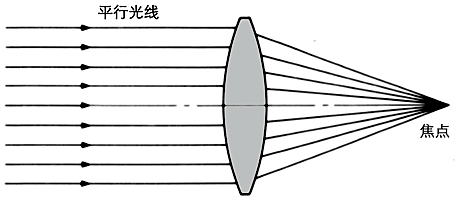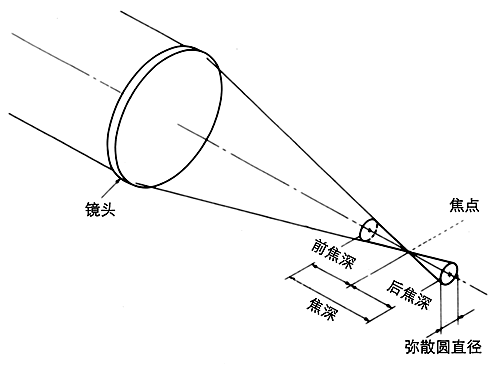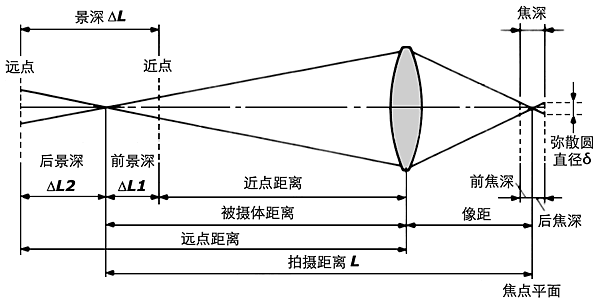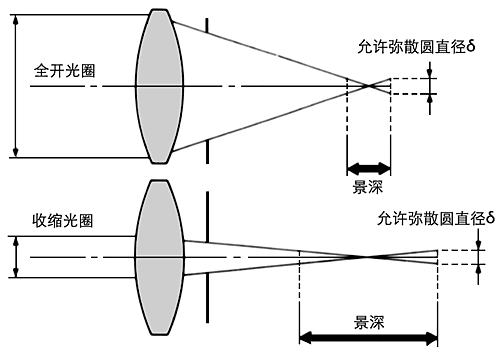# 景深

### 定义

#### 焦点#### 弥散圆#### 景深(depth of field)### 景深的计算

#### 景深的计算公式

• δ：容许弥散圆直径
• f：镜头焦距
• F：镜头光圈
• L：拍摄距离

$ΔL1 = \dfrac{FδL^2}{f^2 + FδL}$

$ΔL1 = \dfrac{FδL^2}{f^2 – FδL}$

$ΔL = ΔL1+ΔL2=\dfrac{2f^2FδL^2}{f^4 – F^2δ^2L^2}$

1. 光圈越大，景深越小；光圈越小，景深越大；
2. 镜头焦距越长，景深越小；焦距越短，景深越大；
3. 距离越远，景深越大；距离越近，景深越小。